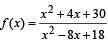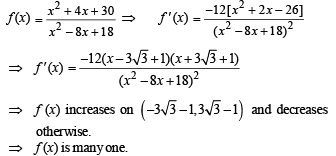Courses

# True/False: Functions | JEE Advanced Notes | EduRev

## JEE : True/False: Functions | JEE Advanced Notes | EduRev

The document True/False: Functions | JEE Advanced Notes | EduRev is a part of the JEE Course Maths 35 Years JEE Main & Advanced Past year Papers.
All you need of JEE at this link: JEE

Q. 1. If f(x) = (a –xn)1/n where a > 0 and n is a positive integer, then f[f(x)] = x.

Ans. T

Solution. f (x) = (a – xn)1/n, a > 0, n is + veinteger
f (f (x)) = f [(a – xn)1/n] = [a –{(a – xn)1/n}n]1/n
= (a – a +  xn)1/n = x

Q. 2. The functionis not one -to -one.

Ans. T

Solution. KEY CONCEPT :  A function is one-one if it is strictly increasing or strictly decreasing, other wise it is many one.Q. 3. If f1(x) and f2(x) are defined on domains D1 and D2 respectively, then f1 (x) + f2 (x) is defined on D1 ∪ D2.

Ans. F

Solution. We know that sum of any two functions is defined only on the points where both f1 as well as f2 are defined that is f1 + f2 is defined on D1 ∩ D2.
∴ The given statement is false.

Offer running on EduRev: Apply code STAYHOME200 to get INR 200 off on our premium plan EduRev Infinity!

## Maths 35 Years JEE Main & Advanced Past year Papers

111 docs|70 tests

,

,

,

,

,

,

,

,

,

,

,

,

,

,

,

,

,

,

,

,

,

;